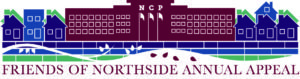## Bring Your Donation to Report Card Pick-Up

Thursday, November 14, 12:00 - 6:00 pm
Stop by the Friends of Northside table just inside the main entrance to make your Annual Appeal donation via cash, check or credit card, and learn more about how you can be an active member of the NCP community. We look forward to talking with you!

## The New Library is Open!

Stop by the library to see first hand how parent donations make a difference to our school. Funds raised at our Spring Benefit in March helped pay for much needed renovations to the the library. Principal Mest will be speaking at 4:15 pm in the library.

Employer donations can double or triple the impact of your donation. That means your \$50 donation can result in up to \$150 for Northside! If you aren't sure if your company matches employee contributions, contact your human resources department.

All donations are tax-deductible, and any amount is greatly appreciated!

 table div table+table+table+table+table div table{width:100%;padding:0}table div table+table+table+table+table div table img{width:96.23%;padding:0;float:none}table div table+table+table+table+table div table td{width:100%;padding:0 1.88% 18px}/* styles */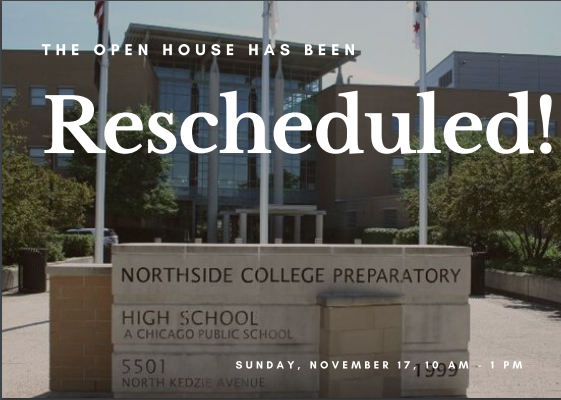# Northside's Open House

## Volunteers Needed!

New date: Sunday, November 17
10:00 am - 1:00 pm

The Northside College Prep Open House has been rescheduled for Sunday, November 17 from 10:00 am - 1:00 pm.

We are in need of many adult volunteers to work this busy but super-fun event. Parents help answer questions and direct crowds in the gym, outside and all over the building. Please click HERE to learn about CPS Volunteer Requirements.

## Volunteers Needed!

Open House Packet Stuffing
Friday, November 15
Help assemble Open House info packets to hand out to prospective families during Open House. Come and go anytime between 8:00-11:00 am & 1:00-4:00 pm as any and all help is welcome. Sign up HERE.

Mock Tour Participants
Friday, November 15
Serve as a mock Open House participant and give tips to students (speak loudly, to your audience, eye contact, etc) during Flex 2 (8:45-9:45 am) or Flex 4 (2:00-3:00 pm) block to help students practice their routes or answer typical tour questions. Sign up HERE.

 table div table+table+table+table+table+table+table+table div table{width:100%;padding:0}table div table+table+table+table+table+table+table+table div table img{width:96.23%;padding:0;float:none}table div table+table+table+table+table+table+table+table div table td{width:100%;padding:0 1.88% 18px}/* styles */# This Week in Sports

Come out and support our student athletes!

## Women's Swimming

▪ Saturday, November 16, Varsity IHSA Sectionals, details TBA
 ▪ Saturday, November 16, Varsity IHSA Sectionals, details TBA

# Winter Sports Tryout and Practice Information

## Winter Sports Online Registration is Now Open

All Winter student athletes must have their parents or guardian register them. Also, all students must have a current physical on file. Students need to turn in a copy of their current physical to their coach. Students can not participate in practice or games without a physical. Visit the NCP athletics website HERE and click on the registration tab.

Tryout Dates:

▪ Poms/Competitive Dance – TBA
▪ Men's Bowling – TBA
▪ Women's Basketball – took place on 11/4
▪ Men's Basketball – 11/11 (non-school day, subject to change)
▪ Wrestling (co-op with Von Steuben) – 11/11
▪ Women's Bowling – 11/18
▪ Men's Swimming and Diving – 11/25
▪ Track (Men and Women) – Jan 2020
 ▪ Poms/Competitive Dance – TBA
 ▪ Men's Bowling – TBA
 ▪ Women's Basketball – took place on 11/4
 ▪ Men's Basketball – 11/11 (non-school day, subject to change)
 ▪ Wrestling (co-op with Von Steuben) – 11/11
 ▪ Women's Bowling – 11/18
 ▪ Men's Swimming and Diving – 11/25
 ▪ Track (Men and Women) – Jan 2020

Visit the NCP Athletics website for information about Northside teams.

 table div table+table+table+table+table+table+table+table+table+table div table{width:100%;padding:0}table div table+table+table+table+table+table+table+table+table+table div table img{width:96.23%;padding:0;float:none}table div table+table+table+table+table+table+table+table+table+table div table td{width:100%;padding:0 1.88% 18px}/* styles */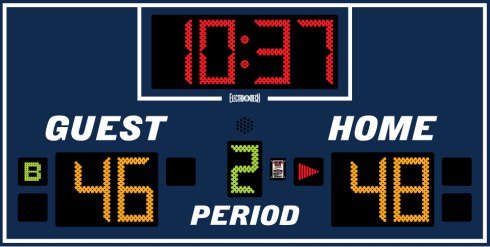# Student or Parent Volunteers Needed for NCP Basketball

Student or parent volunteers are needed to help staff the scorer’s table (operating the new scoreboard and keeping the scorebook) during the regular season and at tournament games for both men's and women's basketball. A small courtesy payment (to come from concessions proceeds) will be offered to interested students. Two volunteers needed per game.

Contact Athletic Director Henry Henderson.

 table div table+table+table+table+table+table+table+table+table+table+table+table div table{width:100%;padding:0}table div table+table+table+table+table+table+table+table+table+table+table+table div table img{width:96.23%;padding:0;float:none}table div table+table+table+table+table+table+table+table+table+table+table+table div table td{width:100%;padding:0 1.88% 18px}/* styles */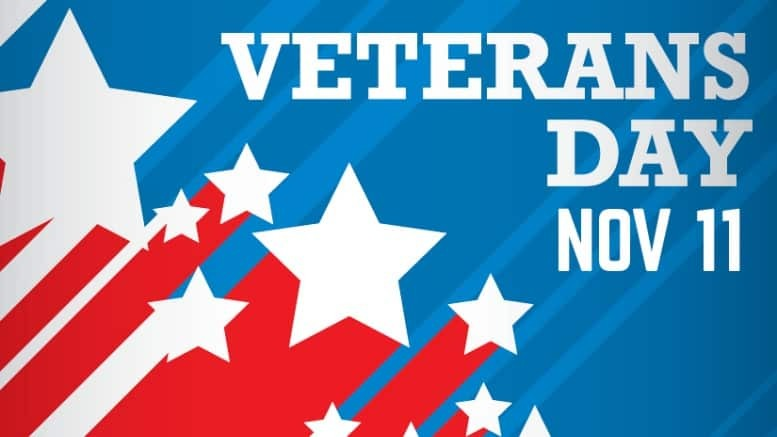# Veteran’s Day

Monday, November 11
No classes for CPS students

 table div table+table+table+table+table+table+table+table+table+table+table+table+table+table div table{width:100%;padding:0}table div table+table+table+table+table+table+table+table+table+table+table+table+table+table div table img{width:96.23%;padding:0;float:none}table div table+table+table+table+table+table+table+table+table+table+table+table+table+table div table td{width:100%;padding:0 1.88% 18px}/* styles */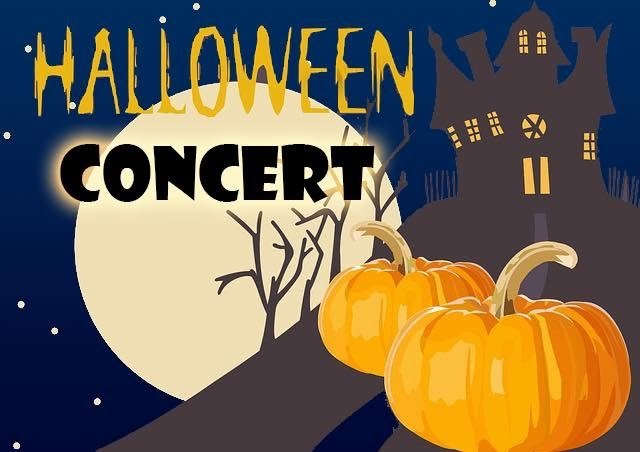# Halloween in November Concert

Tuesday, November 12
7:00 pm
Auditorium

Join the Northside Prep orchestras and choirs as we celebrate Halloween in November

Tickets are \$5 and children 12 and under are free.

 table div table+table+table+table+table+table+table+table+table+table+table+table+table+table+table+table div table{width:100%;padding:0}table div table+table+table+table+table+table+table+table+table+table+table+table+table+table+table+table div table img{width:96.23%;padding:0;float:none}table div table+table+table+table+table+table+table+table+table+table+table+table+table+table+table+table div table td{width:100%;padding:0 1.88% 18px}/* styles */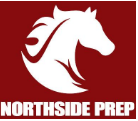# NCP Athletic Boosters Meeting

Wednesday, November 13
7:00 pm
Faculty Lounge

The NCP Athletic Boosters seek to organize support and assistance for our exciting athletic programs, their coaches, and our amazing student-athletes. Please join us to share your ideas and concerns and help us in our quest to increase attendance and student participation at our team events. Get involved and celebrate the incredible achievements of our students outside of the classroom!

All parents and guardians are welcome. Go Mustangs!!!

 table div table+table+table+table+table+table+table+table+table+table+table+table+table+table+table+table+table+table div table{width:100%;padding:0}table div table+table+table+table+table+table+table+table+table+table+table+table+table+table+table+table+table+table div table img{width:96.23%;padding:0;float:none}table div table+table+table+table+table+table+table+table+table+table+table+table+table+table+table+table+table+table div table td{width:100%;padding:0 1.88% 18px}/* styles */# Parent/Teacher Conferences

Thursday, November 14
12:00 - 6:00 pm
Non-attendance Day for Students

Parents can meet with teachers on a first-come, first-served basis or sign up online for 5-minute conferences. You may schedule conferences in advance for any time between 12:00 and 4:00 pm. From 4:00 to 6:00 pm, traditional walk-in appointments will be available.

## Conference Sign-Up

Registration Closes at Midnight on Tuesday, November 12

Online registration for Parent-Teacher conferences is open now and will remain open until midnight Tuesday, November 12. You will have the opportunity to access teachers’ calendars and schedule a convenient conference time.

If signing up online, remember to allow time between conferences to get from one classroom to the next.

# Also on November 14

## Come See the NEW Student Library (made possible by FON fundraising efforts!)

4:15 - 5:15 pm
Library

While you’re at school to meet with your student’s teachers, take advantage of the opportunity to ask questions and participate in a group discussion with Principal Mest.

 table div table+table+table+table+table+table+table+table+table+table+table+table+table+table+table+table+table+table+table+table div table{width:100%;padding:0}table div table+table+table+table+table+table+table+table+table+table+table+table+table+table+table+table+table+table+table+table div table img{width:96.23%;padding:0;float:none}table div table+table+table+table+table+table+table+table+table+table+table+table+table+table+table+table+table+table+table+table div table td{width:100%;padding:0 1.88% 18px}/* styles */# Parent College Night

Thursday, November 14
6:30 pm
Second Floor Atrium

The Friends of Northside sponsored Parent College Night has been rescheduled for Thursday, November 14 at 6:30 pm in the Second Floor Atrium.

Parents of recent Northside graduates will share their experiences, insights, and advice about the college application process. Members of the counseling staff will also be on hand to answer questions.

Please sign-up HERE so we know how many people to expect.

 table div table+table+table+table+table+table+table+table+table+table+table+table+table+table+table+table+table+table+table+table+table+table+table div table{width:100%;padding:0}table div table+table+table+table+table+table+table+table+table+table+table+table+table+table+table+table+table+table+table+table+table+table+table div table img{width:96.23%;padding:0;float:none}table div table+table+table+table+table+table+table+table+table+table+table+table+table+table+table+table+table+table+table+table+table+table+table div table td{width:100%;padding:0 1.88% 18px}/* styles */# DON'T FORGET: AP Exam Registration Deadline Approaching

Register by Friday, November 15

Students taking AP exams at Northside College Prep must register HERE and pay by 4:00 pm on Friday, November 15, 2019.

For the 2020 AP Exams, the cost is \$110 per exam and \$15 if your student qualifies for a free/reduced lunch. Please adhere to the November 15 deadline as there will be a mandatory College Board late fee of \$40.00 charged in addition to the exam fee. Please contact Mr. Alexander Hughes if you have any additional questions.

 table div table+table+table+table+table+table+table+table+table+table+table+table+table+table+table+table+table+table+table+table+table+table+table+table+table+table div table{width:100%;padding:0}table div table+table+table+table+table+table+table+table+table+table+table+table+table+table+table+table+table+table+table+table+table+table+table+table+table+table div table img{width:96.23%;padding:0;float:none}table div table+table+table+table+table+table+table+table+table+table+table+table+table+table+table+table+table+table+table+table+table+table+table+table+table+table div table td{width:100%;padding:0 1.88% 18px}/* styles */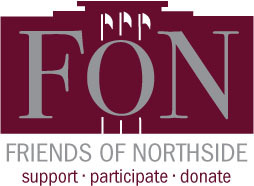# Friends of Northside Meeting

Tuesday, November 19
7:00 pm
Conference Room

Friends of Northside (FON) is the “one-stop-shop” for NCP families to get involved. FON facilitates communication between parents and the school, organizes parent volunteers, plans and sponsors social/educational events, and raises much-needed funds to bridge the gap due to CPS budget cuts. FON supports the school’s mission to maintain and enhance the learning experience for all students of Northside College Prep High School.

To find out more about Friends of Northside, please visit their website HERE.

 table div table+table+table+table+table+table+table+table+table+table+table+table+table+table+table+table+table+table+table+table+table+table+table+table+table+table+table+table div table{width:100%;padding:0}table div table+table+table+table+table+table+table+table+table+table+table+table+table+table+table+table+table+table+table+table+table+table+table+table+table+table+table+table div table img{width:96.23%;padding:0;float:none}table div table+table+table+table+table+table+table+table+table+table+table+table+table+table+table+table+table+table+table+table+table+table+table+table+table+table+table+table div table td{width:100%;padding:0 1.88% 18px}/* styles */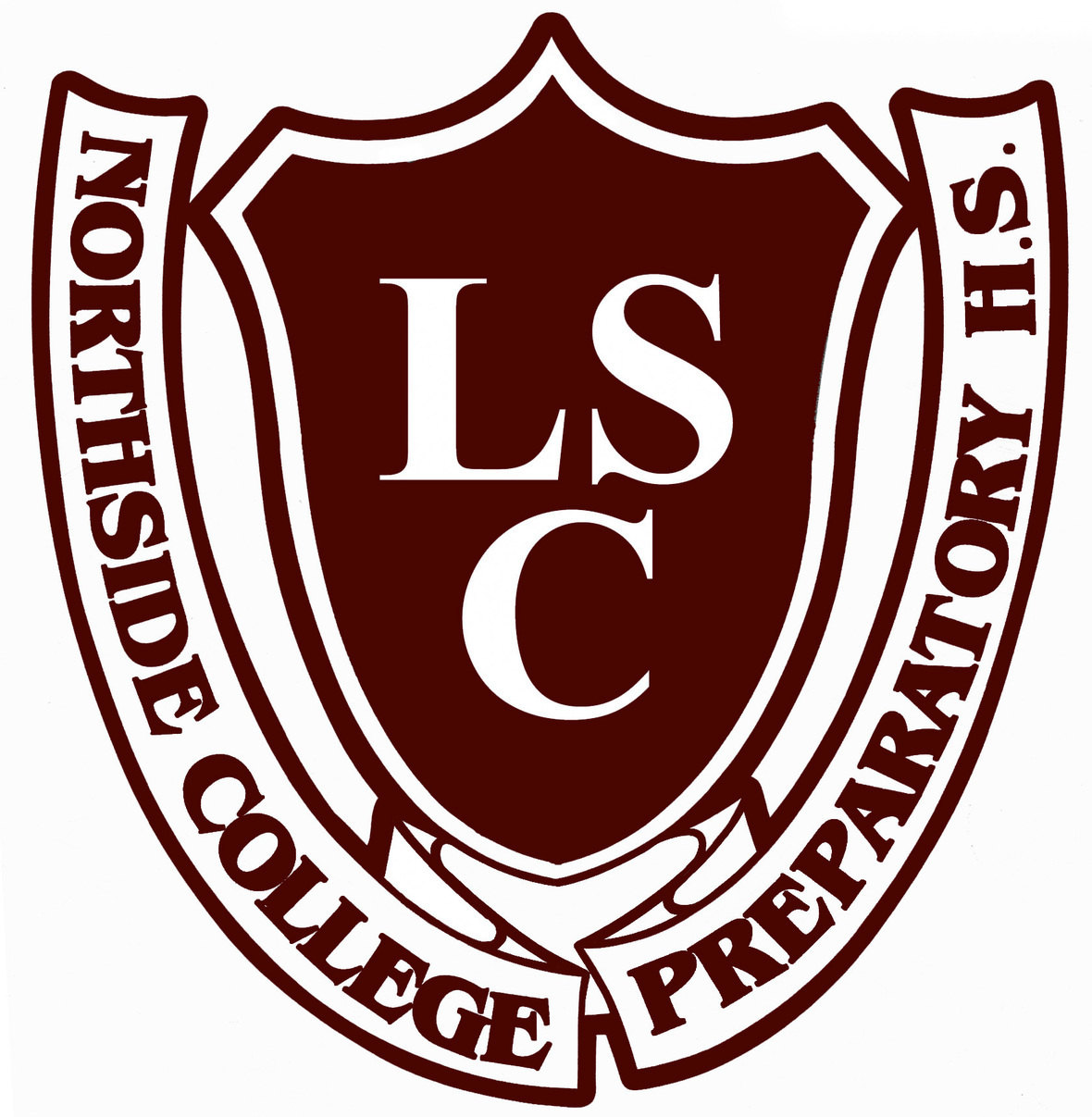# Local School Council (LSC) Meeting

Tuesday, November 19
6:30 pm
Faculty Lounge

The Local School Council (LSC) - the voice in the continuing evolution of Northside - is an elected body that plays an important role in the decision-making that affects our school. The LSC is responsible for approving the Continuous Improvement Work Plan (CIWP), principal selection and evaluation, and allocating discretionary funds to school programs.

LSC elections for six parent and two community representatives will be held in April 2020. Please attend upcoming LSC meetings to get a sense of what NCP’s Local School Council is working on this year.

 table div table+table+table+table+table+table+table+table+table+table+table+table+table+table+table+table+table+table+table+table+table+table+table+table+table+table+table+table+table+table div table{width:100%;padding:0}table div table+table+table+table+table+table+table+table+table+table+table+table+table+table+table+table+table+table+table+table+table+table+table+table+table+table+table+table+table+table div table img{width:96.23%;padding:0;float:none}table div table+table+table+table+table+table+table+table+table+table+table+table+table+table+table+table+table+table+table+table+table+table+table+table+table+table+table+table+table+table div table td{width:100%;padding:0 1.88% 18px}/* styles */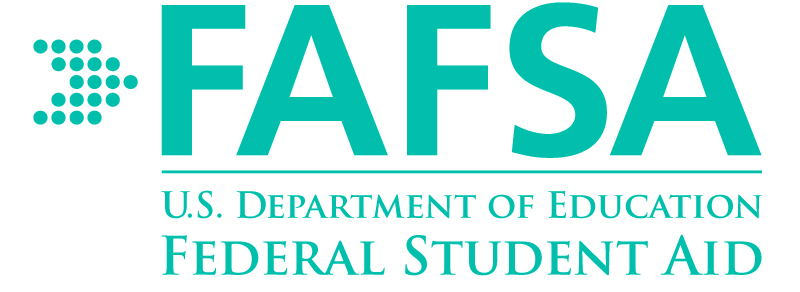# Financial Aid Workshops

## CSS Profile Overview and FAFSA Workshop

Wednesday, November 20
Two sessions: 1:25-2:10 pm and 2:15-3:00 pm
Room 224

## ISAC Student Financial Aid Follow-up

Wednesday, November 20
Two sessions: 1:25-2:10 pm and 2:15-3:00 pm
Room 224

To sign-up for the financial aid workshops, students should login to their Enriching Students portal to register for the sessions. Parents register for workshops HERE.

If you would like to request an individual meeting or workshop after school, please contact Ms. Moore at mcmoore6@cps.edu.

The financial workshops will provide an overview of the College Scholarship Service (CSS) Profile in order to address best practices for navigating need-based financial aid. Assistance will also be provided in completing the FAFSA application. The workshops are only recommended for the class of 2020 students and parents only. Participants should bring the following information:

▪ FSA or College Board login credentials
▪ 2018 and 2019 federal income taxes and asset documentation
▪ W-2 forms and other records of money earned from 2018 and 2019
▪ Records of untaxed income and benefits 2018 and 2019
▪ Records of savings, stocks, bonds, trusts, 529 plans, and other investments
▪ Current bank statements and mortgage information (if applicable, for the CSS Profile only)
▪ The student's noncustodial parent's e-mail address (if applicable, for the CSS Profile only)
 ▪ FSA or College Board login credentials
 ▪ 2018 and 2019 federal income taxes and asset documentation
 ▪ W-2 forms and other records of money earned from 2018 and 2019
 ▪ Records of untaxed income and benefits 2018 and 2019
 ▪ Records of savings, stocks, bonds, trusts, 529 plans, and other investments
 ▪ Current bank statements and mortgage information (if applicable, for the CSS Profile only)
 ▪ The student's noncustodial parent's e-mail address (if applicable, for the CSS Profile only)

ISAC Financial Aid Follow-up Presentations will address what happens after the FAFSA application is completed. Some of the topics will include reviewing the Student Aid Report (SAR), completing the federal income verification process, and using the IRS Data Retrieval Tool.

In addition to the FAFSA Completion workshops, the counseling department has shared some useful resources that may assist you and your family with navigating the next steps in the financial aid process. Click on the titles below to access the FAFSA resources:

▪ The FAFSA Process Graphic by the Federal Student Aid.
▪ 7 Things You Need Before You Fill Out the 2020–21 FAFSA® Form, (2019) by the Federal Student Aid.
▪ FAFSA Tips & Common Mistakes to Avoid (2018) by the National Association of Student Financial Aid Administrators.
▪ 8 Things to Do After Filing Your FAFSA Form (2019) by Chicago Public Schools Office of School Counseling and Postsecondary Advising and the Office of College and Career Success.
 ▪ The FAFSA Process Graphic by the Federal Student Aid.
 ▪ 7 Things You Need Before You Fill Out the 2020–21 FAFSA® Form, (2019) by the Federal Student Aid.
 ▪ FAFSA Tips & Common Mistakes to Avoid (2018) by the National Association of Student Financial Aid Administrators.
 ▪ 8 Things to Do After Filing Your FAFSA Form (2019) by Chicago Public Schools Office of School Counseling and Postsecondary Advising and the Office of College and Career Success.
 table div table+table+table+table+table+table+table+table+table+table+table+table+table+table+table+table+table+table+table+table+table+table+table+table+table+table+table+table+table+table+table+table+table div table{width:100%;padding:0}table div table+table+table+table+table+table+table+table+table+table+table+table+table+table+table+table+table+table+table+table+table+table+table+table+table+table+table+table+table+table+table+table+table div table img{width:96.23%;padding:0;float:none}table div table+table+table+table+table+table+table+table+table+table+table+table+table+table+table+table+table+table+table+table+table+table+table+table+table+table+table+table+table+table+table+table+table div table td{width:100%;padding:0 1.88% 18px}/* styles */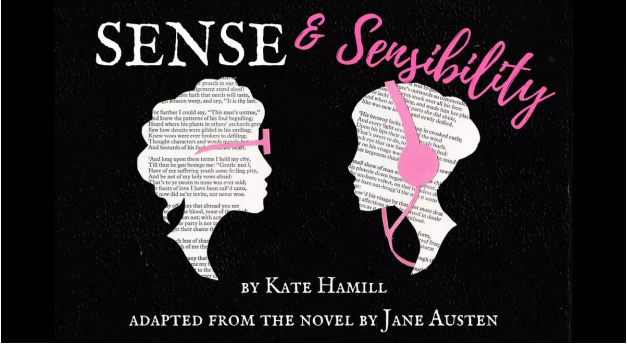# NCP Fall Play: Sense & Sensibility

Thursday, November 21 at 7:00 pm
Friday, November 22 at 7:00 pm
Saturday, November 23 at 7:00 pm
Sunday, November 24 at 3:00 pm

A playful adaptation of Jane Austen’s beloved novel follows the fortunes (and misfortunes) of the Dashwood sisters—sensible Elinor and hypersensitive Marianne—after their father’s sudden death leaves them financially destitute and socially vulnerable. Set in gossipy late 18th-century England with a fresh female voice, the play is full of humor, emotional depth, and bold theatricality. SENSE AND SENSIBILITY examines our reactions, both reasonable and ridiculous, to societal pressures. When reputation is everything, how do you follow your heart?

 table div table+table+table+table+table+table+table+table+table+table+table+table+table+table+table+table+table+table+table+table+table+table+table+table+table+table+table+table+table+table+table+table+table+table+table+table div table{width:100%;padding:0}table div table+table+table+table+table+table+table+table+table+table+table+table+table+table+table+table+table+table+table+table+table+table+table+table+table+table+table+table+table+table+table+table+table+table+table+table div table img{width:96.23%;padding:0;float:none}table div table+table+table+table+table+table+table+table+table+table+table+table+table+table+table+table+table+table+table+table+table+table+table+table+table+table+table+table+table+table+table+table+table+table+table+table div table td{width:100%;padding:0 1.88% 18px}/* styles */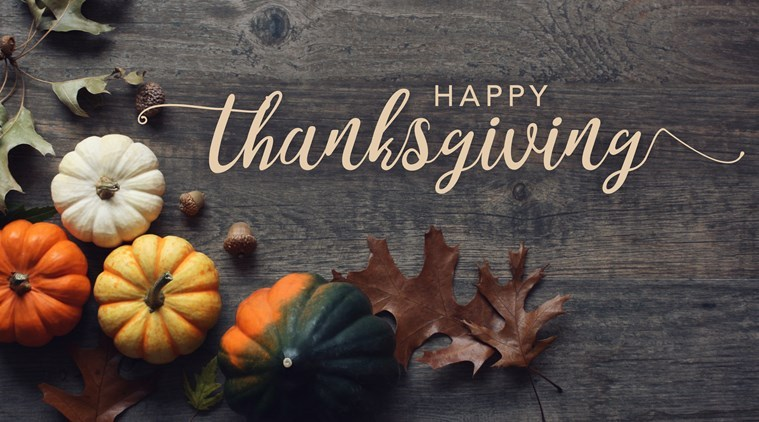# Thanksgiving Break

Wednesday, November 27- (proposed make-up day)
Thursday, November 28
Friday, November 29

 table div table+table+table+table+table+table+table+table+table+table+table+table+table+table+table+table+table+table+table+table+table+table+table+table+table+table+table+table+table+table+table+table+table+table+table+table+table+table div table{width:100%;padding:0}table div table+table+table+table+table+table+table+table+table+table+table+table+table+table+table+table+table+table+table+table+table+table+table+table+table+table+table+table+table+table+table+table+table+table+table+table+table+table div table img{width:96.23%;padding:0;float:none}table div table+table+table+table+table+table+table+table+table+table+table+table+table+table+table+table+table+table+table+table+table+table+table+table+table+table+table+table+table+table+table+table+table+table+table+table+table+table div table td{width:100%;padding:0 1.88% 18px}/* styles */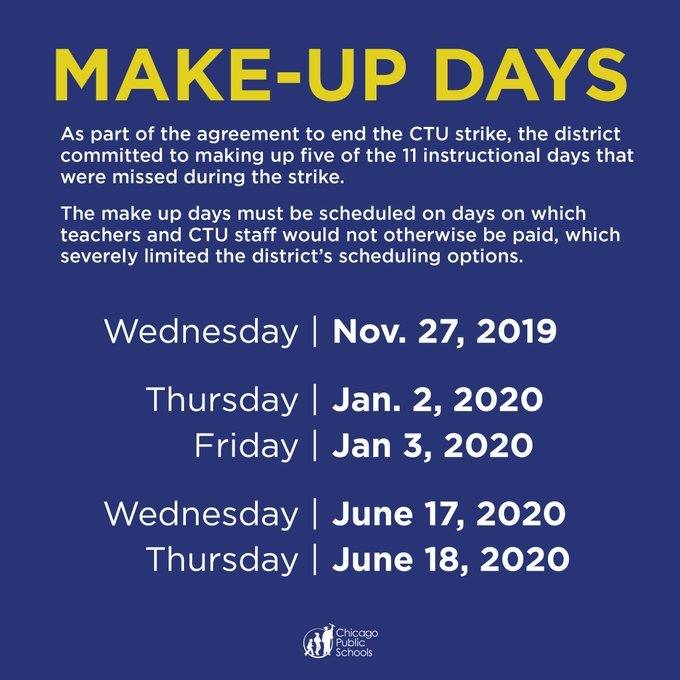# Proposed Make-Up Days

The amended calendar, as well as the district's tentative agreements with both the CTU and SEIU Local 73, will be voted on by the Board of Education at its meeting on November 20. Prior to that vote, the board is planning to hold public hearings on November 12 from 4:00-6:00 pm and 6:30-8:30 pm to collect feedback from members of the community.

 table div table+table+table+table+table+table+table+table+table+table+table+table+table+table+table+table+table+table+table+table+table+table+table+table+table+table+table+table+table+table+table+table+table+table+table+table+table+table+table+table+table div table{width:100%;padding:0}table div table+table+table+table+table+table+table+table+table+table+table+table+table+table+table+table+table+table+table+table+table+table+table+table+table+table+table+table+table+table+table+table+table+table+table+table+table+table+table+table+table div table img{width:96.23%;padding:0;float:none}table div table+table+table+table+table+table+table+table+table+table+table+table+table+table+table+table+table+table+table+table+table+table+table+table+table+table+table+table+table+table+table+table+table+table+table+table+table+table+table+table+table div table td{width:100%;padding:0 1.88% 18px}/* styles */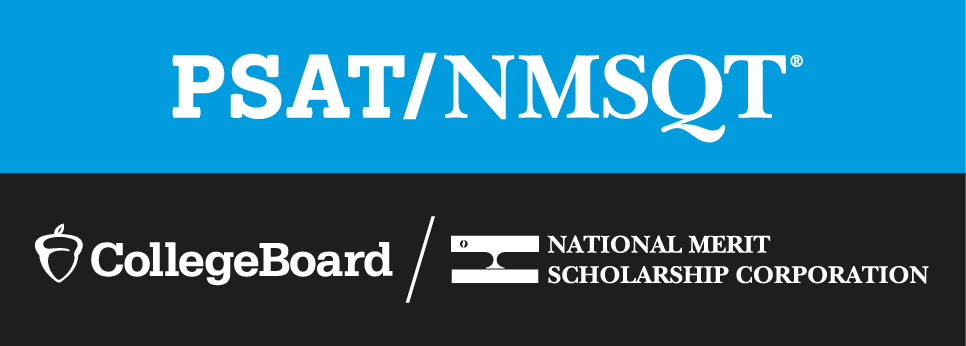# PSAT/NMSQT Update

## 11th Grade Students Will be Able to Submit Their April 2020 SAT Test Scores for Consideration for the National Merit Scholarship

Due to the CTU strike, the PSAT/SAT for sophomores and juniors scheduled for Wednesday, October 30, was cancelled. CPS has confirmation from the College Board and the National Merit Scholarship Corporation that juniors will be able to submit their April 2020 SAT test scores for consideration for the National Merit Scholarship.

 table div table+table+table+table+table+table+table+table+table+table+table+table+table+table+table+table+table+table+table+table+table+table+table+table+table+table+table+table+table+table+table+table+table+table+table+table+table+table+table+table+table+table+table+table div table{width:100%;padding:0}table div table+table+table+table+table+table+table+table+table+table+table+table+table+table+table+table+table+table+table+table+table+table+table+table+table+table+table+table+table+table+table+table+table+table+table+table+table+table+table+table+table+table+table+table div table img{width:96.23%;padding:0;float:none}table div table+table+table+table+table+table+table+table+table+table+table+table+table+table+table+table+table+table+table+table+table+table+table+table+table+table+table+table+table+table+table+table+table+table+table+table+table+table+table+table+table+table+table+table div table td{width:100%;padding:0 1.88% 18px}/* styles */# Travel this Spring with Northside

## Spring Break 2020 Trip to Costa Rica

 table div table+table+table+table+table+table+table+table+table+table+table+table+table+table+table+table+table+table+table+table+table+table+table+table+table+table+table+table+table+table+table+table+table+table+table+table+table+table+table+table+table+table+table+table+table+table div table{width:100%;padding:0}table div table+table+table+table+table+table+table+table+table+table+table+table+table+table+table+table+table+table+table+table+table+table+table+table+table+table+table+table+table+table+table+table+table+table+table+table+table+table+table+table+table+table+table+table+table+table div table img{width:96.23%;padding:0;float:none}table div table+table+table+table+table+table+table+table+table+table+table+table+table+table+table+table+table+table+table+table+table+table+table+table+table+table+table+table+table+table+table+table+table+table+table+table+table+table+table+table+table+table+table+table+table+table div table td{width:100%;padding:0 1.88% 18px}/* styles */# Sculpture Class Looking for Unwanted Chairs

Sculpture II & III students will be transforming chairs into sculptural objects. We are looking for donations of unwanted chairs of any type. Please note, chairs will not be returned. Donations should be left outside of room 111. Chairs are needed as soon as possible. Thanks!

 table div table+table+table+table+table+table+table+table+table+table+table+table+table+table+table+table+table+table+table+table+table+table+table+table+table+table+table+table+table+table+table+table+table+table+table+table+table+table+table+table+table+table+table+table+table+table+table+table div table{width:100%;padding:0}table div table+table+table+table+table+table+table+table+table+table+table+table+table+table+table+table+table+table+table+table+table+table+table+table+table+table+table+table+table+table+table+table+table+table+table+table+table+table+table+table+table+table+table+table+table+table+table+table div table img{width:96.23%;padding:0;float:none}table div table+table+table+table+table+table+table+table+table+table+table+table+table+table+table+table+table+table+table+table+table+table+table+table+table+table+table+table+table+table+table+table+table+table+table+table+table+table+table+table+table+table+table+table+table+table+table+table div table td{width:100%;padding:0 1.88% 18px}/* styles */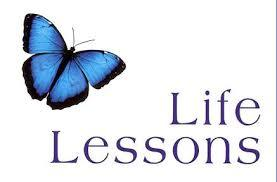# Guest Speakers Needed for Life Lessons Colloquium

Mr. Madia is looking for parents who would like to be a guest speaker for his life lessons colloquium.

He's looking for people in the field of finance, cooking, fashion, travel, hiring manager, auto, housing, life skills, etc. Please contact Mr. Madia directly to help.

 table div table+table+table+table+table+table+table+table+table+table+table+table+table+table+table+table+table+table+table+table+table+table+table+table+table+table+table+table+table+table+table+table+table+table+table+table+table+table+table+table+table+table+table+table+table+table+table+table+table+table div table{width:100%;padding:0}table div table+table+table+table+table+table+table+table+table+table+table+table+table+table+table+table+table+table+table+table+table+table+table+table+table+table+table+table+table+table+table+table+table+table+table+table+table+table+table+table+table+table+table+table+table+table+table+table+table+table div table img{width:96.23%;padding:0;float:none}table div table+table+table+table+table+table+table+table+table+table+table+table+table+table+table+table+table+table+table+table+table+table+table+table+table+table+table+table+table+table+table+table+table+table+table+table+table+table+table+table+table+table+table+table+table+table+table+table+table+table div table td{width:100%;padding:0 1.88% 18px}/* styles */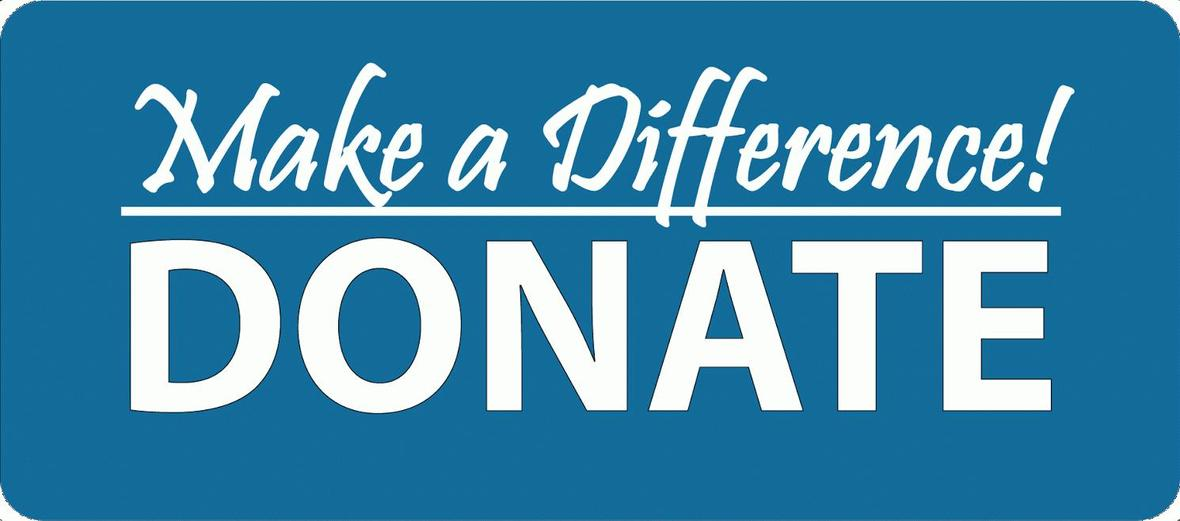# Donations Needed for Student Resource Closet

Friends of Northside has a resource closet available to NCP students. Currently, we are in need of:

▪ Individual snacks such as granola bars, nuts, and dried fruit
▪ Body Lotion
▪ Deodorant for Women
▪ Reusable Water Bottles
▪ Athletic Apparel for Men and Women (shirts, shorts, pants)
▪ Hair conditioner
▪ Men’s and women’s underwear and socks
▪ Mittens and gloves
▪ Sanitizing wipes (Clorox type)
▪ Bath towels
 ▪ Individual snacks such as granola bars, nuts, and dried fruit
 ▪ Body Lotion
 ▪ Deodorant for Women
 ▪ Reusable Water Bottles
 ▪ Athletic Apparel for Men and Women (shirts, shorts, pants)
 ▪ Hair conditioner
 ▪ Men’s and women’s underwear and socks
 ▪ Mittens and gloves
 ▪ Sanitizing wipes (Clorox type)
 ▪ Bath towels

All items must be new and unopened. No used clothing will be accepted. Please bring all items to the Counseling Office and set outside of Ms. Matthews' office.

Contact Nancy Liljedahl with any questions.

 table div table+table+table+table+table+table+table+table+table+table+table+table+table+table+table+table+table+table+table+table+table+table+table+table+table+table+table+table+table+table+table+table+table+table+table+table+table+table+table+table+table+table+table+table+table+table+table+table+table+table+table+table+table div table{width:100%;padding:0}table div table+table+table+table+table+table+table+table+table+table+table+table+table+table+table+table+table+table+table+table+table+table+table+table+table+table+table+table+table+table+table+table+table+table+table+table+table+table+table+table+table+table+table+table+table+table+table+table+table+table+table+table+table div table img{width:96.23%;padding:0;float:none}table div table+table+table+table+table+table+table+table+table+table+table+table+table+table+table+table+table+table+table+table+table+table+table+table+table+table+table+table+table+table+table+table+table+table+table+table+table+table+table+table+table+table+table+table+table+table+table+table+table+table+table+table+table div table td{width:100%;padding:0 1.88% 18px}/* styles */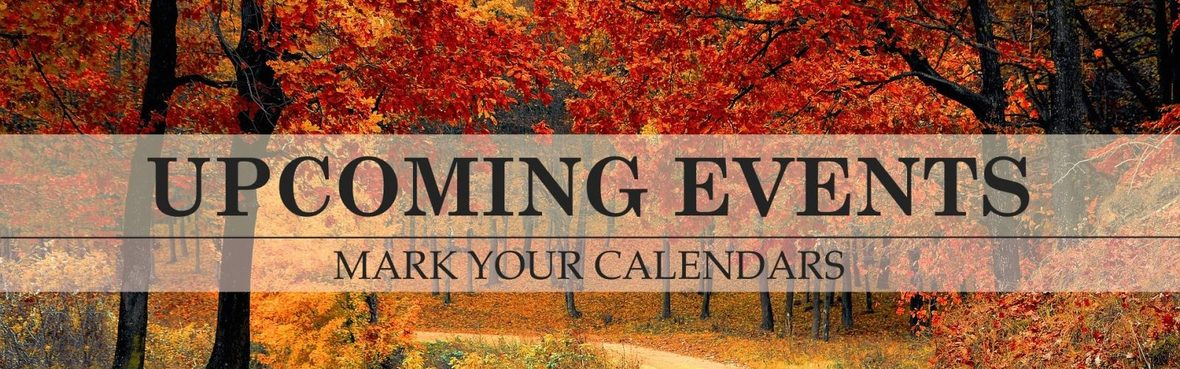# Important November Dates

Please note, these dates are subject to change due to the CTU strike.

▪ Monday, November 11 - No school - Veterans Day
▪ Wednesday, November 13 - Boosters meeting
▪ Thursday, November 14 - No school - Parent-Teacher Conferences
▪ Friday, November 15 - Deadline to register for AP exams
▪ Tuesday, November 19 - LSC meeting and Friends of Northside meeting
▪ Wednesday, November 20 - ISAC Student Financial Aid follow-up
▪ Thursday, November 21 - Sunday, November 24 - Fall Play
▪ Wednesday, November 27 - Friday, November 29 - No school - Fall Break (November 27 is a proposed make-up day)
 ▪ Monday, November 11 - No school - Veterans Day
 ▪ Wednesday, November 13 - Boosters meeting
 ▪ Thursday, November 14 - No school - Parent-Teacher Conferences
 ▪ Friday, November 15 - Deadline to register for AP exams
 ▪ Tuesday, November 19 - LSC meeting and Friends of Northside meeting
 ▪ Wednesday, November 20 - ISAC Student Financial Aid follow-up
 ▪ Thursday, November 21 - Sunday, November 24 - Fall Play
 ▪ Wednesday, November 27 - Friday, November 29 - No school - Fall Break (November 27 is a proposed make-up day)
 table div table+table+table+table+table+table+table+table+table+table+table+table+table+table+table+table+table+table+table+table+table+table+table+table+table+table+table+table+table+table+table+table+table+table+table+table+table+table+table+table+table+table+table+table+table+table+table+table+table+table+table+table+table+table+table+table div table{width:100%;padding:0}table div table+table+table+table+table+table+table+table+table+table+table+table+table+table+table+table+table+table+table+table+table+table+table+table+table+table+table+table+table+table+table+table+table+table+table+table+table+table+table+table+table+table+table+table+table+table+table+table+table+table+table+table+table+table+table+table div table img{width:96.23%;padding:0;float:none}table div table+table+table+table+table+table+table+table+table+table+table+table+table+table+table+table+table+table+table+table+table+table+table+table+table+table+table+table+table+table+table+table+table+table+table+table+table+table+table+table+table+table+table+table+table+table+table+table+table+table+table+table+table+table+table+table div table td{width:100%;padding:0 1.88% 18px}/* styles */# No Experience Necessary

Make a commitment to Northside by offering your time for any of these rewarding on-going volunteer positions. Email Friends of Northside to indicate your interest or ask questions.

## Graphic Designer

Provide professional guidance and materials for various design needs throughout the year.

## Spring Benefit Committee

The Spring Benefit is NCP’s major spring fundraiser. Get involved, get to know NCP staff and parents, and make a profound impact on Northside College Prep.

When is the Spring Benefit?
Friday, March 13, 2020

What’s the time commitment for the team?

Email Debra Swan, Event Chair, for more information or to join the team.

 table div table+table+table+table+table+table+table+table+table+table+table+table+table+table+table+table+table+table+table+table+table+table+table+table+table+table+table+table+table+table+table+table+table+table+table+table+table+table+table+table+table+table+table+table+table+table+table+table+table+table+table+table+table+table+table+table+table+table+table+table div table{width:100%;padding:0}table div table+table+table+table+table+table+table+table+table+table+table+table+table+table+table+table+table+table+table+table+table+table+table+table+table+table+table+table+table+table+table+table+table+table+table+table+table+table+table+table+table+table+table+table+table+table+table+table+table+table+table+table+table+table+table+table+table+table+table+table div table img{width:96.23%;padding:0;float:none}table div table+table+table+table+table+table+table+table+table+table+table+table+table+table+table+table+table+table+table+table+table+table+table+table+table+table+table+table+table+table+table+table+table+table+table+table+table+table+table+table+table+table+table+table+table+table+table+table+table+table+table+table+table+table+table+table+table+table+table+table div table td{width:100%;padding:0 1.88% 18px}/* styles */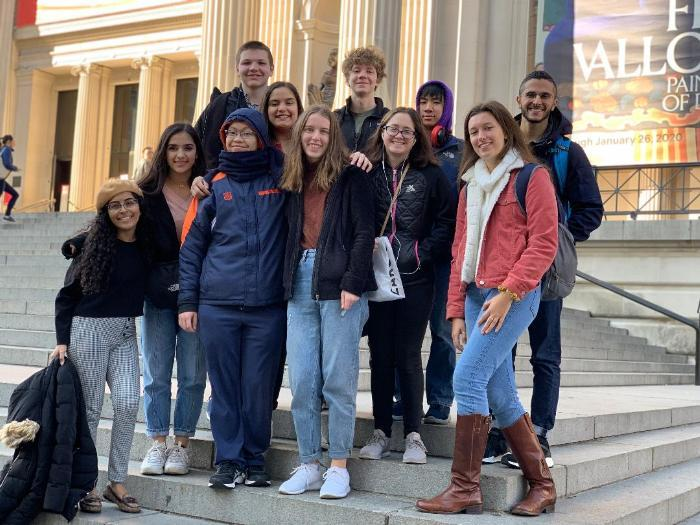# NCP students complete whirlwind trip to 7th Annual Yale Certamen Invitational

A group of nine Northside Latin students traveled to New York City and Yale to compete in Yale's 7th Annual Certamen Invitational. In addition to enjoying the culture and cuisine of New York, they also put forth a valiant effort at the invitational.

Northside's Advanced team of Deena Al-Ali, Matthew Figueras, Gina Jagminas, Richard Lam, and Ruba Memon played very well but ultimately missed out on the semifinals.

Pitted against Boston Latin, the top-scoring team after the first three rounds of competition, and a team from Harriton, NCPers Reem Al-Ali, Joe Giambrone, Gannon Lemaster, and Zoe Okun took the BLS team — the team that went on to win the championship in the next round — to a tie-break round but ultimately lost.

# Do you have news or team results to share with the Northside parent community?

 table div table+table+table+table+table+table+table+table+table+table+table+table+table+table+table+table+table+table+table+table+table+table+table+table+table+table+table+table+table+table+table+table+table+table+table+table+table+table+table+table+table+table+table+table+table+table+table+table+table+table+table+table+table+table+table+table+table+table+table+table+table+table+table+table+table div table{width:100%;padding:0}table div table+table+table+table+table+table+table+table+table+table+table+table+table+table+table+table+table+table+table+table+table+table+table+table+table+table+table+table+table+table+table+table+table+table+table+table+table+table+table+table+table+table+table+table+table+table+table+table+table+table+table+table+table+table+table+table+table+table+table+table+table+table+table+table+table div table img{width:96.23%;padding:0;float:none}table div table+table+table+table+table+table+table+table+table+table+table+table+table+table+table+table+table+table+table+table+table+table+table+table+table+table+table+table+table+table+table+table+table+table+table+table+table+table+table+table+table+table+table+table+table+table+table+table+table+table+table+table+table+table+table+table+table+table+table+table+table+table+table+table+table div table td{width:100%;padding:0 1.88% 18px}/* styles */# November College Visits at Northside

Tuesday, November 12
Ryerson University, 10:38 am
Rutgers University-New Brunswick, 10:38 am

Wednesday, November 13
DePaul University, 9:48 am

Friday, November 15
Northern Illinois University, 3:15 pm

Tuesday, November 19
Indiana Tech, 10:38 am

Monday, November 25
CCC, Harold Washington College, 12:26 pm

Monday, December 2
Lincoln Technical Institute-Chicago, 10:38 am

Tuesday, December 3
National Louis University-Chicago Campus, 11:36 am

The counseling department will reach out to post-secondary representatives to reschedule visits that were unable to take place during the CTU strike.

 table div table+table+table+table+table+table+table+table+table+table+table+table+table+table+table+table+table+table+table+table+table+table+table+table+table+table+table+table+table+table+table+table+table+table+table+table+table+table+table+table+table+table+table+table+table+table+table+table+table+table+table+table+table+table+table+table+table+table+table+table+table+table+table+table+table+table+table+table div table{width:100%;padding:0}table div table+table+table+table+table+table+table+table+table+table+table+table+table+table+table+table+table+table+table+table+table+table+table+table+table+table+table+table+table+table+table+table+table+table+table+table+table+table+table+table+table+table+table+table+table+table+table+table+table+table+table+table+table+table+table+table+table+table+table+table+table+table+table+table+table+table+table+table div table img{width:96.23%;padding:0;float:none}table div table+table+table+table+table+table+table+table+table+table+table+table+table+table+table+table+table+table+table+table+table+table+table+table+table+table+table+table+table+table+table+table+table+table+table+table+table+table+table+table+table+table+table+table+table+table+table+table+table+table+table+table+table+table+table+table+table+table+table+table+table+table+table+table+table+table+table+table div table td{width:100%;padding:0 1.88% 18px}/* styles */# CPS Scholarship Notifications and College Events Around the City

For more details about the upcoming events and to access the most recent updates, view the College Calendar on the Northside Prep Counseling website HERE.

▪ Saturday, November 9 at 9:30 am - Make Your Mark Workshop and Program Kick-Off (For GIRLS aged 13-18 ONLY)
▪ Wednesday, November 13 - App Closes: Jack Kent Cooke College Scholarship
▪ Thursday, November 14 - App Closes: Illinois Professional Land Surveyors Association - Student Scholarships
▪ Saturday, November 16 App Opens: Princeton Prize in Race Relations
▪ Friday, November 22 at 5:30 pm - CPS Student & Parent Scholarship Information Session
▪ Saturday, November 30 - App Closes: Sixt Scholars Program
▪ Sunday, December 1 - App Closes: Dell Scholars Program
▪ Monday, December 2 - App Closes: Greenhouse Scholars Program
▪ Friday, December 6 - App Closes: Comcast Leaders and Achievers Scholarship Program
▪ Monday, December 9 - App Closes: The Science Ambassador Scholarship
 ▪ Saturday, November 9 at 9:30 am - Make Your Mark Workshop and Program Kick-Off (For GIRLS aged 13-18 ONLY)
 ▪ Wednesday, November 13 - App Closes: Jack Kent Cooke College Scholarship
 ▪ Thursday, November 14 - App Closes: Illinois Professional Land Surveyors Association - Student Scholarships
 ▪ Saturday, November 16 App Opens: Princeton Prize in Race Relations
 ▪ Friday, November 22 at 5:30 pm - CPS Student & Parent Scholarship Information Session
 ▪ Saturday, November 30 - App Closes: Sixt Scholars Program
 ▪ Sunday, December 1 - App Closes: Dell Scholars Program
 ▪ Monday, December 2 - App Closes: Greenhouse Scholars Program
 ▪ Friday, December 6 - App Closes: Comcast Leaders and Achievers Scholarship Program
 ▪ Monday, December 9 - App Closes: The Science Ambassador Scholarship
 table div table+table+table+table+table+table+table+table+table+table+table+table+table+table+table+table+table+table+table+table+table+table+table+table+table+table+table+table+table+table+table+table+table+table+table+table+table+table+table+table+table+table+table+table+table+table+table+table+table+table+table+table+table+table+table+table+table+table+table+table+table+table+table+table+table+table+table+table+table+table div table{width:100%;padding:0}table div table+table+table+table+table+table+table+table+table+table+table+table+table+table+table+table+table+table+table+table+table+table+table+table+table+table+table+table+table+table+table+table+table+table+table+table+table+table+table+table+table+table+table+table+table+table+table+table+table+table+table+table+table+table+table+table+table+table+table+table+table+table+table+table+table+table+table+table+table+table div table img{width:96.23%;padding:0;float:none}table div table+table+table+table+table+table+table+table+table+table+table+table+table+table+table+table+table+table+table+table+table+table+table+table+table+table+table+table+table+table+table+table+table+table+table+table+table+table+table+table+table+table+table+table+table+table+table+table+table+table+table+table+table+table+table+table+table+table+table+table+table+table+table+table+table+table+table+table+table+table div table td{width:100%;padding:0 1.88% 18px}/* styles */# New to Parent Portal?

1. Obtain your student's PIN, which should be on your acceptance letter or school welcome letter.
2. Create an account HERE.
 1 Obtain your student's PIN, which should be on your acceptance letter or school welcome letter.
 2 Create an account HERE.

PINs can only be provided to the parent/guardian in person or by mail to the address on file. They cannot be provided by phone or email, or to a student. To request your PIN, please contact Sonia Fernandez in the Counseling Office.

 table div table+table+table+table+table+table+table+table+table+table+table+table+table+table+table+table+table+table+table+table+table+table+table+table+table+table+table+table+table+table+table+table+table+table+table+table+table+table+table+table+table+table+table+table+table+table+table+table+table+table+table+table+table+table+table+table+table+table+table+table+table+table+table+table+table+table+table+table+table+table+table+table div table{width:100%;padding:0}table div table+table+table+table+table+table+table+table+table+table+table+table+table+table+table+table+table+table+table+table+table+table+table+table+table+table+table+table+table+table+table+table+table+table+table+table+table+table+table+table+table+table+table+table+table+table+table+table+table+table+table+table+table+table+table+table+table+table+table+table+table+table+table+table+table+table+table+table+table+table+table+table div table img{width:96.23%;padding:0;float:none}table div table+table+table+table+table+table+table+table+table+table+table+table+table+table+table+table+table+table+table+table+table+table+table+table+table+table+table+table+table+table+table+table+table+table+table+table+table+table+table+table+table+table+table+table+table+table+table+table+table+table+table+table+table+table+table+table+table+table+table+table+table+table+table+table+table+table+table+table+table+table+table+table div table td{width:100%;padding:0 1.88% 18px}/* styles */# CPS Volunteer Requirements

According to CPS policy, anybody who is going to be in contact with students must be approved as a CPS volunteer. There are two levels of volunteers, Level 1 and Level 2.

## Level I Volunteers

(Please allow 8-12 weeks for the entire process)
A fingerprint criminal background check is required for all Level I Volunteers.

A Level I volunteer is:

1. A parent providing volunteer service in their child’s school or classroom for 10 or more hours/week;
2. An individual providing volunteer service for 5 or more hours/week at a school where s/he does not have a child enrolled;
3. Any individual chaperoning an overnight school-sponsored trip, regardless of the hours/week that the volunteer serves (See also the Student Travel Policy).
 1 A parent providing volunteer service in their child’s school or classroom for 10 or more hours/week;
 2 An individual providing volunteer service for 5 or more hours/week at a school where s/he does not have a child enrolled;
 3 Any individual chaperoning an overnight school-sponsored trip, regardless of the hours/week that the volunteer serves (See also the Student Travel Policy).

## Level II Volunteers

(This process can be completed in a day & is required even of volunteers for Friends of Northside events)
A fingerprint criminal background check is not required for Level II Volunteers.

A Level II Volunteer is:

1. A parent providing supervised volunteer service in their child’s school or classroom for less than 10 hours/week;
2. Any individual providing volunteer service for less than 5 hours/week at a school where s/he does not have a child enrolled;
3. An individual providing incidental volunteer service with no ongoing individualized interaction with a student(s) including those who speak at a class/assembly, judge academic competitions, give musical performance, participate in the “Principal for a Day” program, job shadowing event or other one-time event provided where there is direct supervision of the activity/event by regular school employees;
4. A parent accompanying his/her child’s class on a one-day field trip or another type of extracurricular activity that does not involve an overnight stay;
5. An individual providing volunteer service on projects/activities involving no or nominal contact with children (home-based volunteer activities, volunteers serving at Central or Network office).
 1 A parent providing supervised volunteer service in their child’s school or classroom for less than 10 hours/week;
 2 Any individual providing volunteer service for less than 5 hours/week at a school where s/he does not have a child enrolled;
 3 An individual providing incidental volunteer service with no ongoing individualized interaction with a student(s) including those who speak at a class/assembly, judge academic competitions, give musical performance, participate in the “Principal for a Day” program, job shadowing event or other one-time event provided where there is direct supervision of the activity/event by regular school employees;
 4 A parent accompanying his/her child’s class on a one-day field trip or another type of extracurricular activity that does not involve an overnight stay;
 5 An individual providing volunteer service on projects/activities involving no or nominal contact with children (home-based volunteer activities, volunteers serving at Central or Network office).

To become a volunteer, please visit CPS' Volunteer website and follow the instructions. There is also a link on Northside's website on the Parents page Northside Volunteers.

Assistant Principal, Kaitlin O'Hare is Northside's Volunteer Coordinator. After applying to become a volunteer, you must visit the school and show Ms. O'Hare your ID, so she can approve you to move forward in the volunteer process. For Level II volunteers, that is the only step you must take. For Level 1, you will receive further instructions from CPS.

 table div table+table+table+table+table+table+table+table+table+table+table+table+table+table+table+table+table+table+table+table+table+table+table+table+table+table+table+table+table+table+table+table+table+table+table+table+table+table+table+table+table+table+table+table+table+table+table+table+table+table+table+table+table+table+table+table+table+table+table+table+table+table+table+table+table+table+table+table+table+table+table+table+table+table div table{width:100%;padding:0}table div table+table+table+table+table+table+table+table+table+table+table+table+table+table+table+table+table+table+table+table+table+table+table+table+table+table+table+table+table+table+table+table+table+table+table+table+table+table+table+table+table+table+table+table+table+table+table+table+table+table+table+table+table+table+table+table+table+table+table+table+table+table+table+table+table+table+table+table+table+table+table+table+table+table div table img{width:96.23%;padding:0;float:none}table div table+table+table+table+table+table+table+table+table+table+table+table+table+table+table+table+table+table+table+table+table+table+table+table+table+table+table+table+table+table+table+table+table+table+table+table+table+table+table+table+table+table+table+table+table+table+table+table+table+table+table+table+table+table+table+table+table+table+table+table+table+table+table+table+table+table+table+table+table+table+table+table+table+table div table td{width:100%;padding:0 1.88% 18px}/* styles */# FON Connect

Friends of Northside uses a customer/constituent relationship management (CRM) system known as FON Connect that allows our parent volunteer organization to work from one database for events. It is not a CPS database and is completely different and separate from the CPS Parent Portal and Northside's EPay website.

FON Connect also serves as the NCP Family Directory and allows users to review their donation history, update their contact information, register for upcoming events, and more. Each parent has a separate account. Transactions, including donations, are recorded in the account of the parent who initiated the transaction or donation.

If you’re new to FON Connect, you'll need to set up a login and password. To do so:

 table div table+table+table+table+table+table+table+table+table+table+table+table+table+table+table+table+table+table+table+table+table+table+table+table+table+table+table+table+table+table+table+table+table+table+table+table+table+table+table+table+table+table+table+table+table+table+table+table+table+table+table+table+table+table+table+table+table+table+table+table+table+table+table+table+table+table+table+table+table+table+table+table+table+table+table+table div table{width:100%;padding:0}table div table+table+table+table+table+table+table+table+table+table+table+table+table+table+table+table+table+table+table+table+table+table+table+table+table+table+table+table+table+table+table+table+table+table+table+table+table+table+table+table+table+table+table+table+table+table+table+table+table+table+table+table+table+table+table+table+table+table+table+table+table+table+table+table+table+table+table+table+table+table+table+table+table+table+table+table div table img{width:96.23%;padding:0;float:none}table div table+table+table+table+table+table+table+table+table+table+table+table+table+table+table+table+table+table+table+table+table+table+table+table+table+table+table+table+table+table+table+table+table+table+table+table+table+table+table+table+table+table+table+table+table+table+table+table+table+table+table+table+table+table+table+table+table+table+table+table+table+table+table+table+table+table+table+table+table+table+table+table+table+table+table+table div table td{width:100%;padding:0 1.88% 18px}/* styles */# Parking Lot Safety

Our NCP school parking lot is a bustling place before and after school. Families dropping off and picking up students are asked to be mindful of both safety and courtesy. Please assist us by ensuring that traffic lanes stay clear during drop-off and pick-up times and by following the parking lot procedures.

# Parking Lot Procedures

ALL vehicles MUST exit onto Kedzie. You may enter the parking lot from Kedzie or Bryn Mawr, but regardless of where you enter, all cars must exit onto Kedzie. For the safety of our students and the rest of the Northside community, please do not exit onto Bryn Mawr.

The first few days will be busy at drop-off and pick-up. Please, for the safety of all involved, plan to give yourself enough time for drop-off and pick-up and please be patient while in the parking lot. Pulling over to the curb on the right as your student enters or exits your vehicle keeps students safe and traffic flow efficient. Our safety team will be actively working to ensure that students arrive and depart safely each day and that vehicles in school lots remain damage-free.

 table div table+table+table+table+table+table+table+table+table+table+table+table+table+table+table+table+table+table+table+table+table+table+table+table+table+table+table+table+table+table+table+table+table+table+table+table+table+table+table+table+table+table+table+table+table+table+table+table+table+table+table+table+table+table+table+table+table+table+table+table+table+table+table+table+table+table+table+table+table+table+table+table+table+table+table+table+table+table div table{width:100%;padding:0}table div table+table+table+table+table+table+table+table+table+table+table+table+table+table+table+table+table+table+table+table+table+table+table+table+table+table+table+table+table+table+table+table+table+table+table+table+table+table+table+table+table+table+table+table+table+table+table+table+table+table+table+table+table+table+table+table+table+table+table+table+table+table+table+table+table+table+table+table+table+table+table+table+table+table+table+table+table+table div table img{width:96.23%;padding:0;float:none}table div table+table+table+table+table+table+table+table+table+table+table+table+table+table+table+table+table+table+table+table+table+table+table+table+table+table+table+table+table+table+table+table+table+table+table+table+table+table+table+table+table+table+table+table+table+table+table+table+table+table+table+table+table+table+table+table+table+table+table+table+table+table+table+table+table+table+table+table+table+table+table+table+table+table+table+table+table+table div table td{width:100%;padding:0 1.88% 18px}/* styles */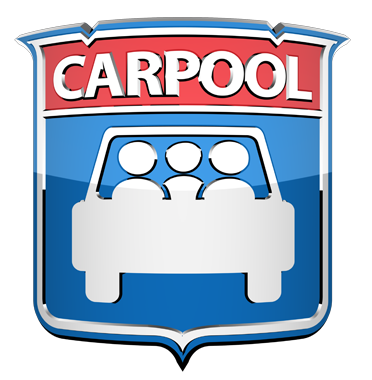# NCP Carpool Connection!

If you're interested in carpooling to school, add your address to the 2019-2020 NCP Carpool map and reach out to contact a potential carpool family on the map. Find the map HERE or on the Welcome page of FON Connect.

 table div table+table+table+table+table+table+table+table+table+table+table+table+table+table+table+table+table+table+table+table+table+table+table+table+table+table+table+table+table+table+table+table+table+table+table+table+table+table+table+table+table+table+table+table+table+table+table+table+table+table+table+table+table+table+table+table+table+table+table+table+table+table+table+table+table+table+table+table+table+table+table+table+table+table+table+table+table+table+table+table div table{width:100%;padding:0}table div table+table+table+table+table+table+table+table+table+table+table+table+table+table+table+table+table+table+table+table+table+table+table+table+table+table+table+table+table+table+table+table+table+table+table+table+table+table+table+table+table+table+table+table+table+table+table+table+table+table+table+table+table+table+table+table+table+table+table+table+table+table+table+table+table+table+table+table+table+table+table+table+table+table+table+table+table+table+table+table div table img{width:96.23%;padding:0;float:none}table div table+table+table+table+table+table+table+table+table+table+table+table+table+table+table+table+table+table+table+table+table+table+table+table+table+table+table+table+table+table+table+table+table+table+table+table+table+table+table+table+table+table+table+table+table+table+table+table+table+table+table+table+table+table+table+table+table+table+table+table+table+table+table+table+table+table+table+table+table+table+table+table+table+table+table+table+table+table+table+table div table td{width:100%;padding:0 1.88% 18px}/* styles */# Attention, Parents of CTA Commuters

Did you know that the #82 and #92 buses pick up students in front of Northside at the end of the school day? The Kimball Brown line station is also close to school at Kimball and Lawrence.

These are just a few of the ways students can use public transportation to commute to school. More information on routes for your student can be found at the CTA website HERE.

 table div table+table+table+table+table+table+table+table+table+table+table+table+table+table+table+table+table+table+table+table+table+table+table+table+table+table+table+table+table+table+table+table+table+table+table+table+table+table+table+table+table+table+table+table+table+table+table+table+table+table+table+table+table+table+table+table+table+table+table+table+table+table+table+table+table+table+table+table+table+table+table+table+table+table+table+table+table+table+table+table+table+table div table{width:100%;padding:0}table div table+table+table+table+table+table+table+table+table+table+table+table+table+table+table+table+table+table+table+table+table+table+table+table+table+table+table+table+table+table+table+table+table+table+table+table+table+table+table+table+table+table+table+table+table+table+table+table+table+table+table+table+table+table+table+table+table+table+table+table+table+table+table+table+table+table+table+table+table+table+table+table+table+table+table+table+table+table+table+table+table+table div table img{width:96.23%;padding:0;float:none}table div table+table+table+table+table+table+table+table+table+table+table+table+table+table+table+table+table+table+table+table+table+table+table+table+table+table+table+table+table+table+table+table+table+table+table+table+table+table+table+table+table+table+table+table+table+table+table+table+table+table+table+table+table+table+table+table+table+table+table+table+table+table+table+table+table+table+table+table+table+table+table+table+table+table+table+table+table+table+table+table+table+table div table td{width:100%;padding:0 1.88% 18px}/* styles */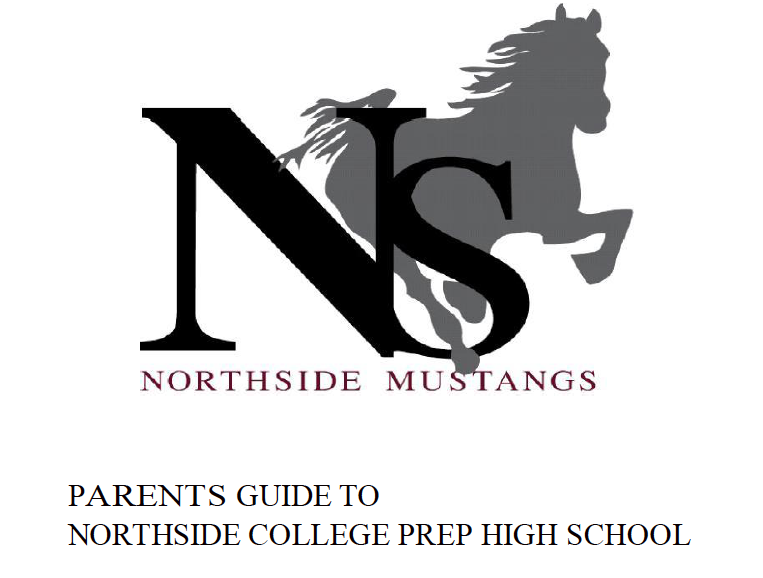# Navigating Northside

Navigating Northside, our parent handbook, is available on the school website under the "Parents" tab. Click HERE to access it directly.

 table div table+table+table+table+table+table+table+table+table+table+table+table+table+table+table+table+table+table+table+table+table+table+table+table+table+table+table+table+table+table+table+table+table+table+table+table+table+table+table+table+table+table+table+table+table+table+table+table+table+table+table+table+table+table+table+table+table+table+table+table+table+table+table+table+table+table+table+table+table+table+table+table+table+table+table+table+table+table+table+table+table+table+table+table div table{width:100%;padding:0}table div table+table+table+table+table+table+table+table+table+table+table+table+table+table+table+table+table+table+table+table+table+table+table+table+table+table+table+table+table+table+table+table+table+table+table+table+table+table+table+table+table+table+table+table+table+table+table+table+table+table+table+table+table+table+table+table+table+table+table+table+table+table+table+table+table+table+table+table+table+table+table+table+table+table+table+table+table+table+table+table+table+table+table+table div table img{width:96.23%;padding:0;float:none}table div table+table+table+table+table+table+table+table+table+table+table+table+table+table+table+table+table+table+table+table+table+table+table+table+table+table+table+table+table+table+table+table+table+table+table+table+table+table+table+table+table+table+table+table+table+table+table+table+table+table+table+table+table+table+table+table+table+table+table+table+table+table+table+table+table+table+table+table+table+table+table+table+table+table+table+table+table+table+table+table+table+table+table+table div table td{width:100%;padding:0 1.88% 18px}/* styles */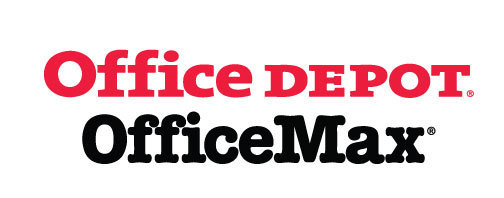# Give Back to Northside

Purchase school and office supplies at Office Depot and OfficeMax and Northside can receive 5% back in credits or FREE supplies. Simply provide our school ID 70031171 at checkout, online, or in stores. There’s no cost to you!

 table div table+table+table+table+table+table+table+table+table+table+table+table+table+table+table+table+table+table+table+table+table+table+table+table+table+table+table+table+table+table+table+table+table+table+table+table+table+table+table+table+table+table+table+table+table+table+table+table+table+table+table+table+table+table+table+table+table+table+table+table+table+table+table+table+table+table+table+table+table+table+table+table+table+table+table+table+table+table+table+table+table+table+table+table+table+table div table{width:100%;padding:0}table div table+table+table+table+table+table+table+table+table+table+table+table+table+table+table+table+table+table+table+table+table+table+table+table+table+table+table+table+table+table+table+table+table+table+table+table+table+table+table+table+table+table+table+table+table+table+table+table+table+table+table+table+table+table+table+table+table+table+table+table+table+table+table+table+table+table+table+table+table+table+table+table+table+table+table+table+table+table+table+table+table+table+table+table+table+table div table img{width:96.23%;padding:0;float:none}table div table+table+table+table+table+table+table+table+table+table+table+table+table+table+table+table+table+table+table+table+table+table+table+table+table+table+table+table+table+table+table+table+table+table+table+table+table+table+table+table+table+table+table+table+table+table+table+table+table+table+table+table+table+table+table+table+table+table+table+table+table+table+table+table+table+table+table+table+table+table+table+table+table+table+table+table+table+table+table+table+table+table+table+table+table+table div table td{width:100%;padding:0 1.88% 18px}/* styles */# Support Northside - Shop AmazonSmile

AmazonSmile is a simple and automatic way to support Northside every time you shop.

Please note that our AmazonSmile name is Friends of "North" "Side" (North Side - two words at AmazonSmile).

 table div table+table+table+table+table+table+table+table+table+table+table+table+table+table+table+table+table+table+table+table+table+table+table+table+table+table+table+table+table+table+table+table+table+table+table+table+table+table+table+table+table+table+table+table+table+table+table+table+table+table+table+table+table+table+table+table+table+table+table+table+table+table+table+table+table+table+table+table+table+table+table+table+table+table+table+table+table+table+table+table+table+table+table+table+table+table+table+table div table{width:100%;padding:0}table div table+table+table+table+table+table+table+table+table+table+table+table+table+table+table+table+table+table+table+table+table+table+table+table+table+table+table+table+table+table+table+table+table+table+table+table+table+table+table+table+table+table+table+table+table+table+table+table+table+table+table+table+table+table+table+table+table+table+table+table+table+table+table+table+table+table+table+table+table+table+table+table+table+table+table+table+table+table+table+table+table+table+table+table+table+table+table+table div table img{width:96.23%;padding:0;float:none}table div table+table+table+table+table+table+table+table+table+table+table+table+table+table+table+table+table+table+table+table+table+table+table+table+table+table+table+table+table+table+table+table+table+table+table+table+table+table+table+table+table+table+table+table+table+table+table+table+table+table+table+table+table+table+table+table+table+table+table+table+table+table+table+table+table+table+table+table+table+table+table+table+table+table+table+table+table+table+table+table+table+table+table+table+table+table+table+table div table td{width:100%;padding:0 1.88% 18px}/* styles */# Follow Us on Social Media

▪ Northside Prep Parents Facebook Page, a closed group for parents, by parents, to share their knowledge of Northside
▪ Friends of Northside Facebook Page, for news everyone can view
 ▪ Northside Prep Parents Facebook Page, a closed group for parents, by parents, to share their knowledge of Northside
 ▪ Friends of Northside Facebook Page, for news everyone can view
 table div table+table+table+table+table+table+table+table+table+table+table+table+table+table+table+table+table+table+table+table+table+table+table+table+table+table+table+table+table+table+table+table+table+table+table+table+table+table+table+table+table+table+table+table+table+table+table+table+table+table+table+table+table+table+table+table+table+table+table+table+table+table+table+table+table+table+table+table+table+table+table+table+table+table+table+table+table+table+table+table+table+table+table+table+table+table+table+table+table+table div table{width:100%;padding:0}table div table+table+table+table+table+table+table+table+table+table+table+table+table+table+table+table+table+table+table+table+table+table+table+table+table+table+table+table+table+table+table+table+table+table+table+table+table+table+table+table+table+table+table+table+table+table+table+table+table+table+table+table+table+table+table+table+table+table+table+table+table+table+table+table+table+table+table+table+table+table+table+table+table+table+table+table+table+table+table+table+table+table+table+table+table+table+table+table+table+table div table img{width:96.23%;padding:0;float:none}table div table+table+table+table+table+table+table+table+table+table+table+table+table+table+table+table+table+table+table+table+table+table+table+table+table+table+table+table+table+table+table+table+table+table+table+table+table+table+table+table+table+table+table+table+table+table+table+table+table+table+table+table+table+table+table+table+table+table+table+table+table+table+table+table+table+table+table+table+table+table+table+table+table+table+table+table+table+table+table+table+table+table+table+table+table+table+table+table+table+table div table td{width:100%;padding:0 1.88% 18px}/* styles */Northside’s website can be found HERE.

Friends of Northside's website can be found HERE.

 table div table+table+table+table+table+table+table+table+table+table+table+table+table+table+table+table+table+table+table+table+table+table+table+table+table+table+table+table+table+table+table+table+table+table+table+table+table+table+table+table+table+table+table+table+table+table+table+table+table+table+table+table+table+table+table+table+table+table+table+table+table+table+table+table+table+table+table+table+table+table+table+table+table+table+table+table+table+table+table+table+table+table+table+table+table+table+table+table+table+table+table+table div table{width:100%;padding:0}table div table+table+table+table+table+table+table+table+table+table+table+table+table+table+table+table+table+table+table+table+table+table+table+table+table+table+table+table+table+table+table+table+table+table+table+table+table+table+table+table+table+table+table+table+table+table+table+table+table+table+table+table+table+table+table+table+table+table+table+table+table+table+table+table+table+table+table+table+table+table+table+table+table+table+table+table+table+table+table+table+table+table+table+table+table+table+table+table+table+table+table+table div table img{width:96.23%;padding:0;float:none}table div table+table+table+table+table+table+table+table+table+table+table+table+table+table+table+table+table+table+table+table+table+table+table+table+table+table+table+table+table+table+table+table+table+table+table+table+table+table+table+table+table+table+table+table+table+table+table+table+table+table+table+table+table+table+table+table+table+table+table+table+table+table+table+table+table+table+table+table+table+table+table+table+table+table+table+table+table+table+table+table+table+table+table+table+table+table+table+table+table+table+table+table div table td{width:100%;padding:0 1.88% 18px}/* styles */